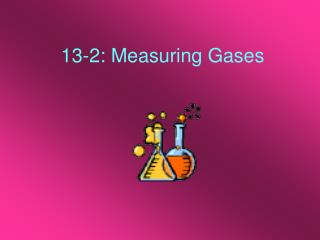DownloadDownload Presentation13-2: Measuring Gases

# 13-2: Measuring Gases

Download Presentation## 13-2: Measuring Gases

- - - - - - - - - - - - - - - - - - - - - - - - - - - E N D - - - - - - - - - - - - - - - - - - - - - - - - - - -
##### Presentation Transcript

1. 13-2: Measuring Gases

2. Variables • There are four different variables to consider when measuring gases: • Amount of Gas: [n] usually measured in moles. • Volume: [V] measured in liters [l]. • Temperature: [T] measured in Kelvins [K] K = C + 273 4. Pressure: [P] measured in a variety of units [atmospheres, torr, psi, pascals, mmHg]

3. Atmospheric Pressure • Atmospheric pressure: • The pressure exerted by air in the atmosphere. • Results from the fact that the air has mass and is attracted to the Earth by gravity. 1atm = 101,325 Pa 1kPa = 1000 Pa

4. Pressure (cont.) • Altimeter: a device that converts the atmospheric pressure according to altitude. • Since water vapor is “lighter” than the nitrogen and oxygen gas, the lower the atmospheric pressure, the more water vapor present. H20 = 18.02 g/mol 02 = 32.00 g/mol N2 = 28.02 g/mol

5. Pressure (cont.) • Barometer: device used to measure pressure of the atmosphere, often measured in mmHg. • Manometer: device used to measure the pressure of a gas in an enclosed container. • STP [Standard Temperature and Pressure]: 273 K and 1 atm Jr

6. Important Conversion Factors • 1 atm = 101,325 Pa • 1kPa = 1000 Pa • 1 atm = 760 mmHg = 760 torr • 1 atm = 14.70 psi • 1 bar = 100,000 Pa = 0.9869 atm

7. The End Hope you learned a lot!